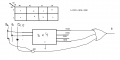# Is there any other way more clever to implement this boolean function using a 2*4 decoder?

#### asdasd12e12

Joined Nov 24, 2021
19
So here is the function and my attempt:Where the ' annotation means complement and Q2 is the most significant bit and Q0 the least significant bit.

I'm also trying to implement the same function but this time using only one 1*2 decoder but without much sucess. If the only entry of the decoder is Q2, pretty much most of the circuit is just using and's and or's gates without really much of the actual decoder.

#### ApacheKid

Joined Jan 12, 2015
617
So here is the function and my attempt:

View attachment 256934

Where the ' annotation means complement and Q2 is the most significant bit and Q0 the least significant bit.

I'm also trying to implement the same function but this time using only one 1*2 decoder but without much sucess. If the only entry of the decoder is Q2, pretty much most of the circuit is just using and's and or's gates without really much of the actual decoder.
I can't make sense of that diagram.

How many inputs does this have?

How many outputs?

Can you just list a table of every possible input and the desired output for each case?